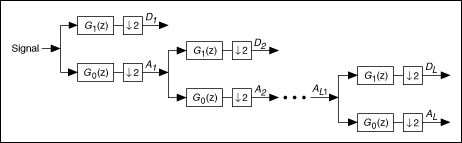# Discrete Wavelet Transform (Advanced Signal Processing Toolkit)

LabVIEW 2014 Advanced Signal Processing Toolkit Help

Edition Date: June 2014

Part Number: 372656C-01

»View Product InfoDownload Help (Windows Only)

Unlike the discrete Fourier transform (DFT), which is a discrete version of the Fourier transform, the discrete wavelet transform (DWT) is not really a discrete version of the continuous wavelet transform (CWT). Instead, the DWT is functionally different from the CWT. To implement the DWT, you use discrete filter banks to compute discrete wavelet coefficients. Two-channel perfect reconstruction (PR) filter banks are a common and efficient way to implement the DWT. The following figure shows a typical two-channel PR filter bank system.The signal X(z) first is filtered by a filter bank consisting of G0(z) and G1(z). The outputs of G0(z) and G1(z) then are downsampled by a factor of 2. After some processing, the modified signals are upsampled by a factor of 2 and filtered by another filter bank consisting of H0(z) and H1(z).

If no processing takes place between the two filter banks, the sum of outputs of H0(z) and H1(z) is identical to the original signal X(z), except for the time delay. This system is a two-channel PR filter bank, where G0(z) and G1(z) form an analysis filter bank, and H0(z) and H1(z) form a synthesis filter bank.

Traditionally, G0(z) and H0(z) are lowpass filters, and G1(z) and H1(z) are highpass filters. The subscripts 0 and 1 represent lowpass and highpass filters, respectively. The operation ↓2 denotes a decimation of the signal by a factor of two. Applying decimation factors to the signal ensures that the number of output samples of the two lowpass filters equal the number of original input samples X(z). Therefore, no redundant information is added during the decomposition. Refer to the LabVIEW Digital Filter Design Toolkit documentation for more information about filters.

You can use the two-channel PR filter bank system and consecutively decompose the outputs of lowpass filters, as shown in the following figure.Lowpass filters remove high-frequency fluctuations from the signal and preserve slow trends. The outputs of lowpass filters provide an approximation of the signal. Highpass filters remove the slow trends from the signal and preserve high-frequency fluctuations. The outputs of highpass filters provide detail information about the signal. The outputs of lowpass filters and highpass filters define the approximation coefficients and detail coefficients, respectively. Symbols A and D in the previous figure represent the approximation and detail information, respectively.

You also can call the detail coefficients wavelet coefficients because detail coefficients approximate the inner products of the signal and wavelets.Note  This help alternately uses the terms wavelet coefficients and detail coefficients, depending on the context.

The LabVIEW Wavelet Analysis Tools use the subscripts 0 and 1 to describe the decomposition path, where 0 indicates lowpass filtering and 1 indicates highpass filtering. For example, D2 in the previous figure denotes the output of two cascaded filtering operations—lowpass filtering followed by highpass filtering. Therefore, you can describe this decomposition path with the sequence 01. Similarly, DL denotes the output of the filtering operations 000...1 in which the total number of 0 is L–1. The impulse response of 000...1 converges asymptotically to the mother wavelet and the impulse response of 000...0 converges to the scaling function in the wavelet transform.

The DWT is invertible, meaning that you can reconstruct the signal from the DWT coefficients with the inverse DWT. The inverse DWT also is implemented with filter banks by cascading the synthesis filter banks. The following figure shows the inverse DWT using filter banks.Use the WA Discrete Wavelet Transform VI to compute the DWT of 1D and 2D signals. Use the WA Inverse Discrete Wavelet Transform VI to compute the inverse DWT of 1D and 2D signals. Use the WA Get Coefficients of Discrete Wavelet Transform VI to get DWT coefficients you compute from the WA Discrete Wavelet Transform VI and to return the coefficient type, such as the approximation coefficients or the detail coefficients, at a specific coefficient level. Use the WA Set Coefficients of Discrete Wavelet Transform VI to set the coefficients you obtain from the WA Get Coefficients of Discrete Wavelet Transform VI.

Refer to the Get and Set Approximation Coefficients VI in the labview\examples\Wavelet Analysis\WAGettingStarted directory for an example of how to get and set the approximation coefficients that you compute from the discrete wavelet transform.

Refer to the Get and Set Single Level Detail Coefficients VI in the labview\examples\Wavelet Analysis\WAGettingStarted directory for an example of how to get and set the detail coefficients that you compute from the discrete wavelet transform.